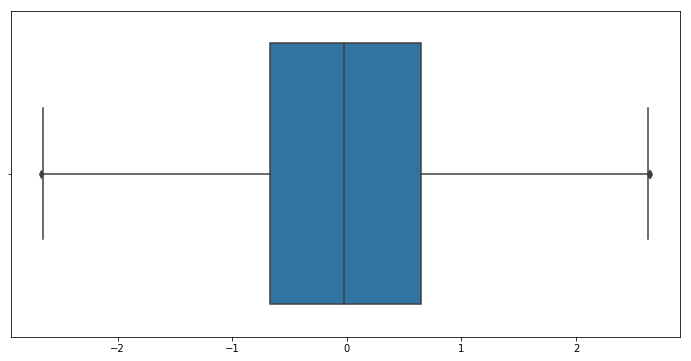# 2.7 - Outlier Detection Using Boxplots

Last updated: April 3rd, 2019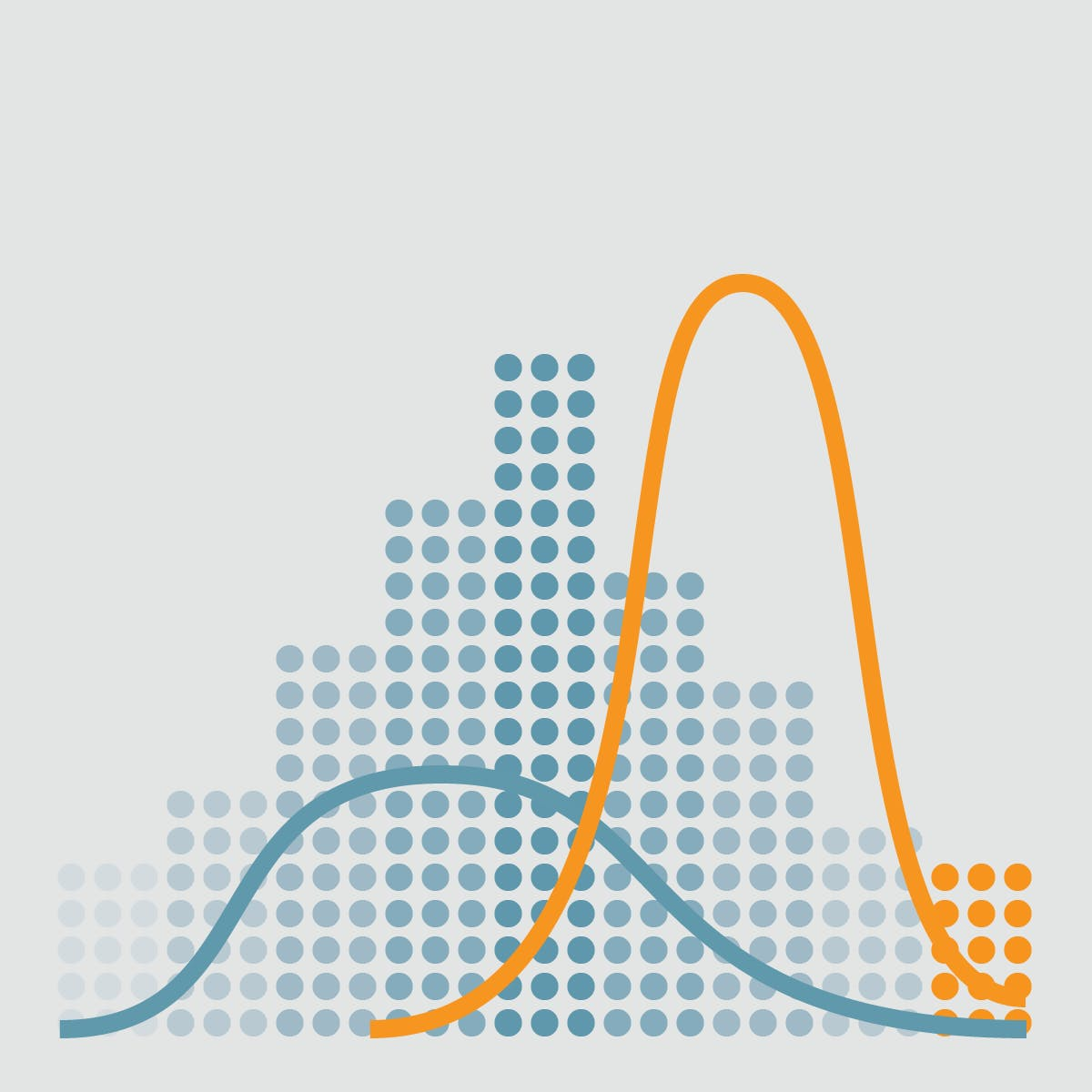# Outlier detection with Boxplots¶

In descriptive statistics, a box plot or boxplot is a method for graphically depicting groups of numerical data through their quartiles. Box plots may also have lines extending vertically from the boxes (whiskers) indicating variability outside the upper and lower quartiles, hence the terms box-and-whisker plot and box-and-whisker diagram.## Hands on!¶

In :
import numpy as np
import pandas as pd
import matplotlib.pyplot as plt
import seaborn as sns

%matplotlib inline### Boxplot¶

A box and whisker plot —also called a box plot— displays five-number summary of a set of data.

Boxplots are a standardized way of displaying the distribution of data based on a five number summary ("minimum", first quartile (Q1), median, third quartile (Q3), and "maximum").

This type of plot is used to easily detect outliers. It can also tell us if your data is symmetrical, how tightly your data is grouped, and if and how your data is skewed.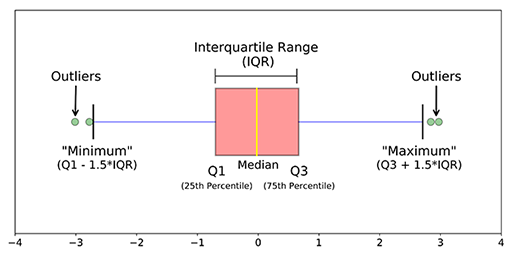• median (Q2/50th Percentile): the middle value of the dataset.
• first quartile (Q1/25th Percentile): the middle number between the smallest number (not the "minimum") and the median of the dataset.
• third quartile (Q3/75th Percentile): the middle value between the median and the highest value (not the "maximum") of the dataset.
• InterQuartile Range (IQR): 25th to the 75th percentile. IQR tells how spread the middle values are.
• "maximum": Q3 + 1.5*IQR
• "minimum": Q1 -1.5*IQR
• Outliers: (shown as green circles) In statistics, an outlier is an observation point that is distant from other observations.

Not every outlier is a wrong value.### Boxplot of a Normal distribution¶

In :
normal = np.random.normal(0, 1, 10000) # loc, scale, size
quartiles = pd.DataFrame(normal).quantile([0.25, 0.5, 0.75, 1])

fig, axs = plt.subplots(nrows=2)
fig.set_size_inches(14, 8)

# Boxplot of Normal distribution
plot1 = sns.boxplot(normal, ax=axs)
plot1.set(xlim=(-4, 4))

# Normal distribution
plot2 = sns.distplot(normal, ax=axs)
plot2.set(xlim=(-4, 4))

# Median line
plt.axvline(np.median(normal), color='#e74c3c', linestyle='dashed', linewidth=2)

for i, q in enumerate(quartiles):
# Quartile i line
plt.axvline(q, color='#27ae60', linestyle='dotted', linewidth=2)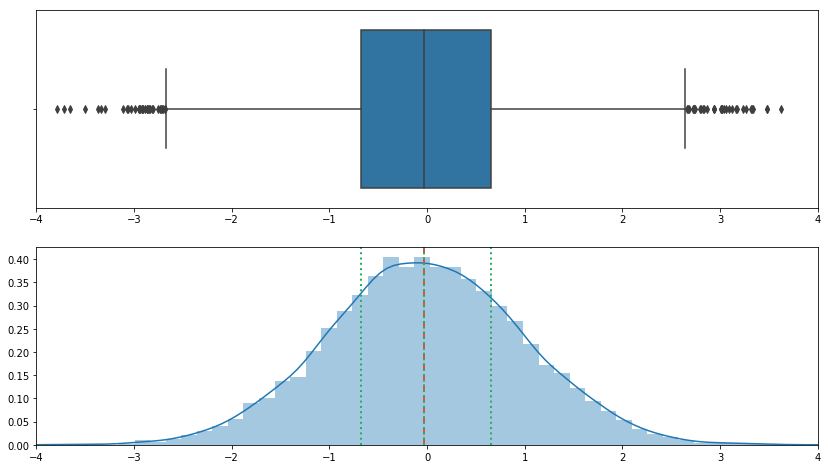### Outliers detection¶

Now that we know how to build a boxplot and visualize outliers (points outside whiskers), lets remove them:

In :
plt.figure(figsize=(12,6))

sns.boxplot(normal)

Out:
<matplotlib.axes._subplots.AxesSubplot at 0x7f929a016b00>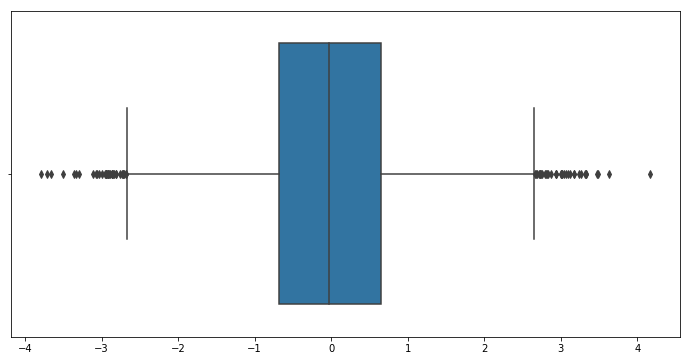Boxplot show us many outliers, but are they wrong values?

We can manually remove values below/above a certain value:

In :


Out:
array([ 0.46258854,  1.03999792, -0.96583145, ...,  1.36174267,
-0.7491783 ,  0.34268328])
In :
plt.figure(figsize=(12,6))

sns.boxplot(normal[(normal >= -3) & (normal <= 3)])

Out:
<matplotlib.axes._subplots.AxesSubplot at 0x7f9299975518>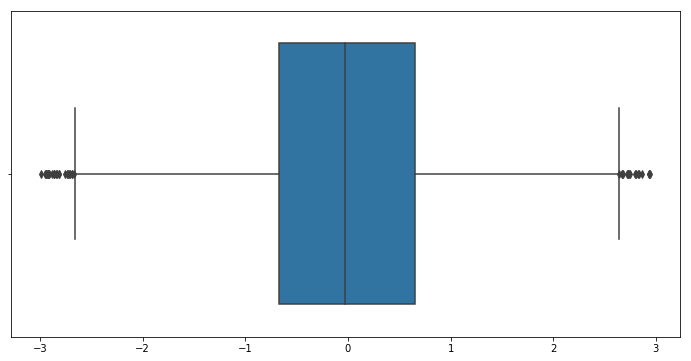Or use low and high fences of the boxplot and remove outer elements:

In :
q1 = pd.DataFrame(normal).quantile(0.25)
q3 = pd.DataFrame(normal).quantile(0.75)
iqr = q3 - q1 #Interquartile range

fence_low = q1 - (1.5*iqr)
fence_high = q3 + (1.5*iqr)

In :
iqr

Out:
1.3323599334949785
In :
fence_low

Out:
-2.676000401933399
In :
fence_high

Out:
2.6534393320465153
In :
# "Outside" boxplot Reviews
normal[(normal < fence_low) | (normal > fence_high)].shape

Out:
70

Keep just the "inside" boxplot points:

In :
plt.figure(figsize=(12,6))

sns.boxplot(normal[(normal >= fence_low) & (normal <= fence_high)])

Out:
<matplotlib.axes._subplots.AxesSubplot at 0x7f929998db00>